# Play music with a speaker

You have tried to produce sound using a buzzer. It sounds indeed so sharp. So this time, you are going to enjoy the music played by the speaker. It provides a higher audio quality.

Learning goals

• Learn how a speaker produces different sounds.
• Understand the difference between buzzer and speaker.
• Have a general idea of I2S protocol.
• Know about different sound waveforms.
• Realize the difference between WAVE and MP3 files.

## 🔸Background​

### What is I2S?​

I2S, short for inter-integrated circuit sound, is specially designed for audio data transmission. It uses three wires for communication:

• SCK (Serial clock): or Bit Clock (BCLK), it carries the clock signal. Its clock frequency equals Sample Rate x Bits per channel x Number of channels.
• FS (Frame Sync): or Word Select (WS), it tells that the audio data being transmitted is for the right or left channel.
• SD (Serial data): it is used to transfer audio data.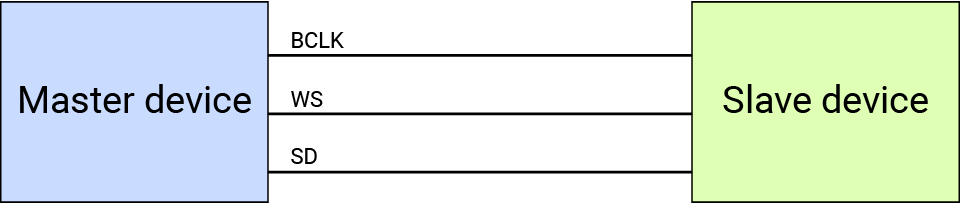The SwiftIO Feather board always serves as a master device. It has two I2S interfaces:

• one is I2SOut. It sends out data to other audio devices, like speakers, so the serial data line is TX, used to send data.
• the other is I2SIn, and the corresponding serial data line (RX) only receives audio data. It can be used with, for example, microphones to collect sound info.

## 🔸New concept​

### Audio​

You listen to music in everyday life, but do you know how audio is stored on your computer? Besides, there are so many different file formats, what’s their difference? Let’s find out more about it.

Waveform

The waveforms of sound are various. Sine, square, triangle, and sawtooth waves are four commonly-used forms. The sounds produced by them are quite different. Random combinations of different waveforms can produce really amazing sounds.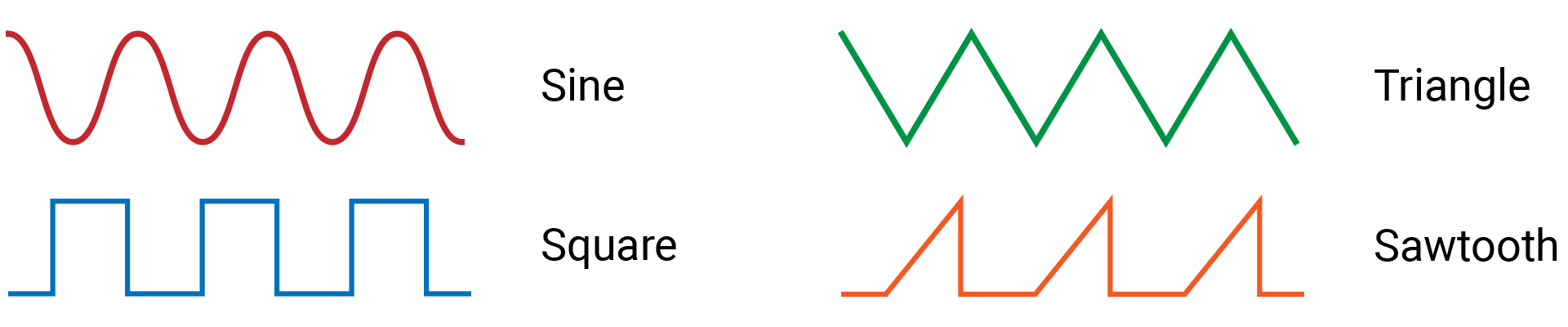We’ll talk about the sine wave in the following part and only deal with periodic waveforms. The sine wave is the fundamental wave. Almost all other period waveforms can be broken down into several different sine waves using Fourier Transforms.

A periodic waveform repeats the wave in a period and produces constant sound. The frequency measures how many times the wave repeats in a second, measured in hertz (hz). For example, the wave below repeats the minimum wave 5 times, so the frequency is 5Hz. The higher the frequency, the higher the pitch. And the frequencies of human hearing are about 20Hz to 20kHz.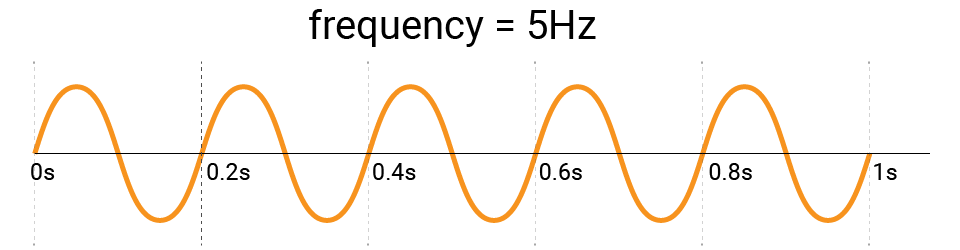Sampling

The audio signal is analog and the data always change with time. There are some sampling techniques to store the data digitally. Let’s take a look at the one called pulse code modulation (PCM). Briefly speaking, it records the sound amplitudes at a regular time interval. Then the samples will be encoded into digital values. As long as the sample data are as many as possible, you could largely recreate the original audio signal.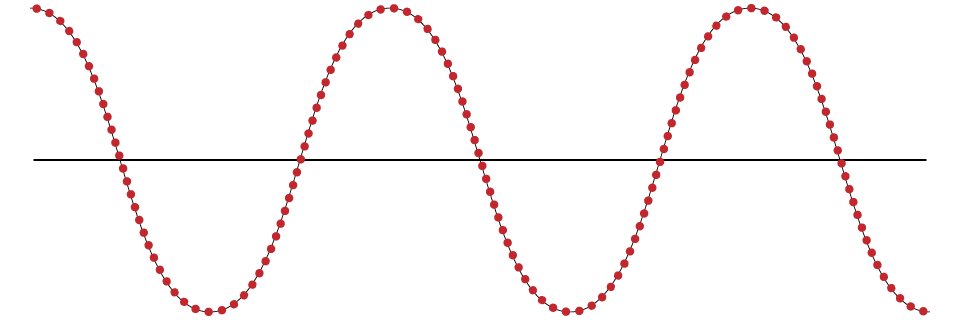Sample rate describes how many times the signal is sampled in one second, measured in hertz (hz). There is a known law about the sample rate: Nyquist rate. It says the sample rate should be more than twice the frequency of the original signal, or it may cause distortion.

Sample depth corresponds to the number of possible values for each sample. The common choices are 16, 24, 32-bit. The higher the sample depth, the more precise the samples can be, so the reproduce sound will be closer to the original one.

When you wear earphones to listen to music, you can hear sounds in your left and right ear. The L and R are used respectively for the left and right channels. If only one channel is sampled, the sound is mono. Stereo means the audio has multiple channels, usually the right and left channel, and thus needs two speakers.

As mentioned before, the maximum frequency for human hearing is about 20KHz, the sample rate should be double to recreate sounds, that is, about 40KHz. The CD audio is usually sampled at 44,100Hz, in other words, 44100 samples per second, which should cover almost all sounds humans can hear. And CD-quality audio usually has 2 channels with a sample depth of 16-bit.

Audio format

Maybe you have heard of some audio file formats: MP3, WAV, AAC, FLAC, and so on. These formats can be divided into two types: lossless and lossy file format. The difference is the way audio data is stored. For example, WAV and FLAC are lossless, while MP3 and AAC are lossy. Let’s have a look at the two frequently-used formats as an example: WAV and MP3.

• WAV (or WAVE) file stores the raw PCM data. Besides, it has a header at the beginning which tells the sampling information. Or else others cannot know how data is sampled. This format stores all original audio data, thus it is large in file size but doesn’t require any decoding method to open the file.

• MP3 files use compression algorithms to remove some of the frequencies that human ears are not sensitive to. So this quality loss is not perceptible to most listeners. Compared to the WAV file, MP3 is much smaller in size, thus it is really handy to download from the Internet. Because it uses compression algorithms, the music player needs to decode it to open the file.

## 🔸New component​

### Speaker​

The working principle of the speaker is similar to the buzzer. When the current flows, the magnet field generated in the circuit cause the internal diaphragm to move back and forth, thus causing the air vibration. The air molecules vibrate and bump into each other, which leads to the sound you hear.

The diaphragm in a buzzer can only move back and forth among determined positions. The different sounds are generated by changing the speed of vibration. However, the diaphragm inside a speaker can move to different positions according to the signal. So a speaker can produce various sounds, and the sound is of higher quality. So it is suitable to reproduce the audio signal it receives and is widely used to play music.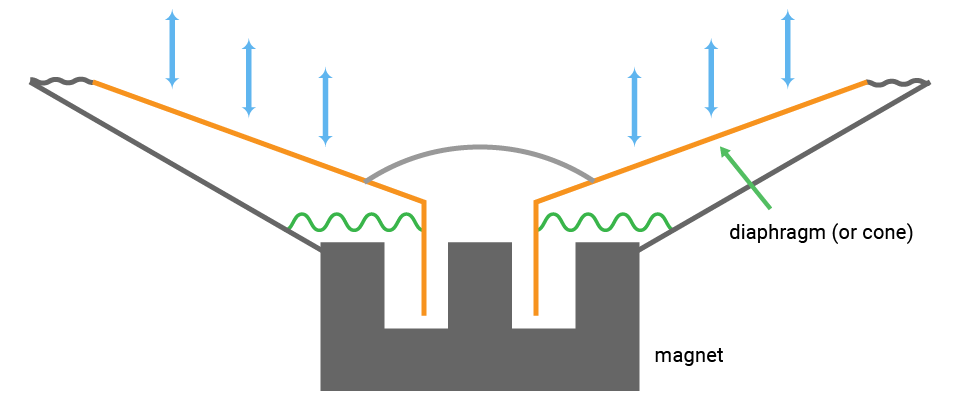Symbol: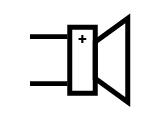The speaker needs an analog signal to produce sounds. But the audio signal transmitted through the I2S bus is a digital signal, which is is not the signal that the speaker requires. Thus there is an additional chip beside the speaker that can convert the digital signal to an analog signal, known as DAC.

The analog signal from the DAC can only drive a headphone and is not strong enough to drive a speaker. So an amplifier is needed to amplify the signal for the speaker.

The chip MAX98357 provides both two functionalities and supports I2S protocol. It has been added to the circuit to allow the speaker to play music.

This chip needs stereo audio and can output audio with the left channel, right channel, or both channels. However, we have configured it to only output left channel data when designing the circuit.

## 🔸Circuit​

The speaker connects to the chip MAX98357. And the chip connects to I2SOut0 (SYNC0, BCLK0, TX0).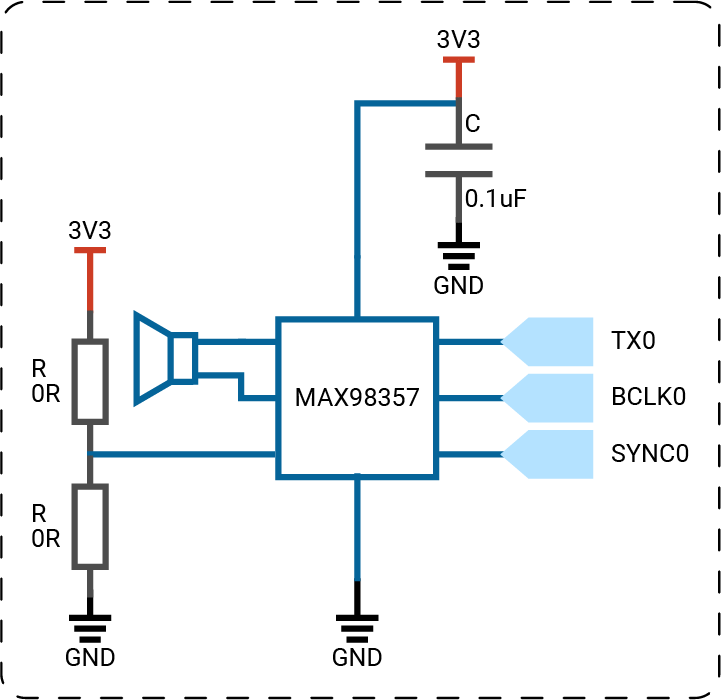note

The circuits above are simplified versions for your reference.

## 🔸Preparation​

Class

`I2SOut` - send audio data to external devices using I2S protocol.

MethodExplanation
`init(_:rate:bits:`
`channel:mode:)`
Initialize an I2S output interface for audio devices.
Parameter:
- `idName`: the specified I2SOut pin.
- `rate`: the sample rate of the audio. The default rate is 16000 Hz.
- `bits`: the sample depth of the audio, 16-bit by default.
- `channel`: the audio channel settings: left, right and stereo. By default, it is `.monoLeft`.
- `mode`: define when the data is sent. The `.philips` is the default mode.
`write(_:count:`
`timeout:`)
Send audio data out to devices.
Parameter:
- `sample`: the audio data stored in a UInt8 array.
- `count`: the count of data to be sent.

## 🔸Projects​

1. Play scales

### 1. Play scales​

Different waveforms can generate different sounds. In this project, you will generate a square wave and a triangle wave manually. Then play scales using two sounds.Example code

C01S09Speaker.swift
``// Import the SwiftIO library to control input and output.import SwiftIO// Import the MadBoard to use the id of the pins.import MadBoard@mainpublic struct C01S09Speaker {    public static func main() {        // Initialize the speaker using I2S communication.         // The default setting is 16k sample rate, 16bit sample bits.        let speaker = I2SOut(Id.I2SOut0)        // The frequencies of note C to B in octave 4.        let frequency: [Float] = [            261.626,            293.665,            329.628,            349.228,            391.995,            440.000,            493.883        ]        // Set the samples of the waveforms.        let sampleRate = 16_000        let rawSampleLength = 1000        var rawSamples = [Int16](repeating: 0, count: rawSampleLength)        var amplitude: Int16 = 10_000        while true {            let duration: Float = 1.0            // Iterate through the frequencies from C to B to play a scale.             // The sound waveform is a square wave, so you will hear a buzzing sound.            generateSquare(amplitude: amplitude, &rawSamples)            for f in frequency {                playWave(samples: rawSamples, frequency: f, duration: duration)            }             sleep(ms: 1000)            // Iterate through the frequencies from C to B to play a scale.            // The sound waveform is a triangle wave, and the sound is much softer.            generateTriangle(amplitude: amplitude, &rawSamples)            for f in frequency {                playWave(samples: rawSamples, frequency: f, duration: duration)            }             sleep(ms: 1000)            // Decrease the amplitude to lower the sound.            // If it's smaller than zero, it restarts from 20000.            amplitude -= 1000            if amplitude <= 0 {                amplitude = 10_000            }        }        // Generate samples for a square wave with a specified amplitude and store them in an array.        func generateSquare(amplitude: Int16, _ samples: inout [Int16]) {            let count = samples.count            for i in 0..<count / 2 {                samples[i] = -amplitude            }            for i in count / 2..<count {                samples[i] = amplitude            }        }        // Generate samples for a triangle wave with a specified amplitude and store the them in an array.        func generateTriangle(amplitude: Int16, _ samples: inout [Int16]) {            let count = samples.count            let step = Float(amplitude) / Float(count / 2)            for i in 0..<count / 4 {                samples[i] = Int16(step * Float(i))            }            for i in count / 4..<count / 4 * 3 {                samples[i] = amplitude - Int16(step * Float(i))            }            for i in count / 4 * 3..<count {                samples[i] = -amplitude + Int16(step * Float(i))            }        }        // Send the samples over I2s bus and play the note with a specified frequency and duration.        func playWave(samples: [Int16], frequency: Float, duration: Float) {            let playCount = Int(duration * Float(sampleRate))            var data = [Int16](repeating: 0, count: playCount)            let step: Float = frequency * Float(samples.count) / Float(sampleRate)            var volume: Float = 1.0            let volumeStep = 1.0 / Float(playCount)            for i in 0..<playCount {                let pos = Int(Float(i) * step) % samples.count                 data[i] = Int16(Float(samples[pos]) * volume)                volume -= volumeStep            }            data.withUnsafeBytes { ptr in                let u8Array = ptr.bindMemory(to: UInt8.self)                speaker.write(Array(u8Array))            }        }    }}``

Code analysis

``import SwiftIOimport MadBoard``

Import the `SwiftIO` library to set I2S communication and the `MadBoard` to use pin ids.

``let speaker = I2SOut(Id.I2SOut0)``

Initialize an `I2SOut` interface reserved for the speaker. It will have a 16k sample rate and 16-bit sample depth by default.

``let frequency: [Float] = [261.626, 293.665, 329.628, 349.228, 391.995, 440.000, 493.883]``

Store frequencies for note C, D, E, F, G, A, B in octave 4. That constitutes a scale, which will be played by the speaker.

``let sampleRate = 16_000let rawSampleLength = 1000var rawSamples = [Int16](repeating: 0, count: rawSampleLength)var amplitude: Int16 = 10_000``

Define the parameters for the audio data:

• The signal is sampled at 16000 Hz, so there will be 16000 data per second.
• `rawSampleLength` decides the count of samples of the generated waves in a period.
• `rawSamples` stores the samples of the audio signal in a period. At first, all values are filled with 0 and the count is decided by `rawSampleLength`.
• `amplitude` is the peak value of the wave and should be positive.
``func generateSquare(amplitude: Int16, _ samples: inout [Int16]) {    let count = samples.count    for i in 0..<count / 2 {        samples[i] = -amplitude    }    for i in count / 2..<count {        samples[i] = amplitude    }}``

This newly defined function allows you to generate a periodic square wave. You only need to calculate the samples in one period. The other periods of waves will repeat these samples. The parameter `samples` needs an array to store the audio data, so it is set as `inout` to be changed inside the function.

A square wave has only two states (0 and 1), so the calculation is quite simple. The first half samples are all negative, and samples of the second half are positive. Their values are all decided by the parameter `amplitude`.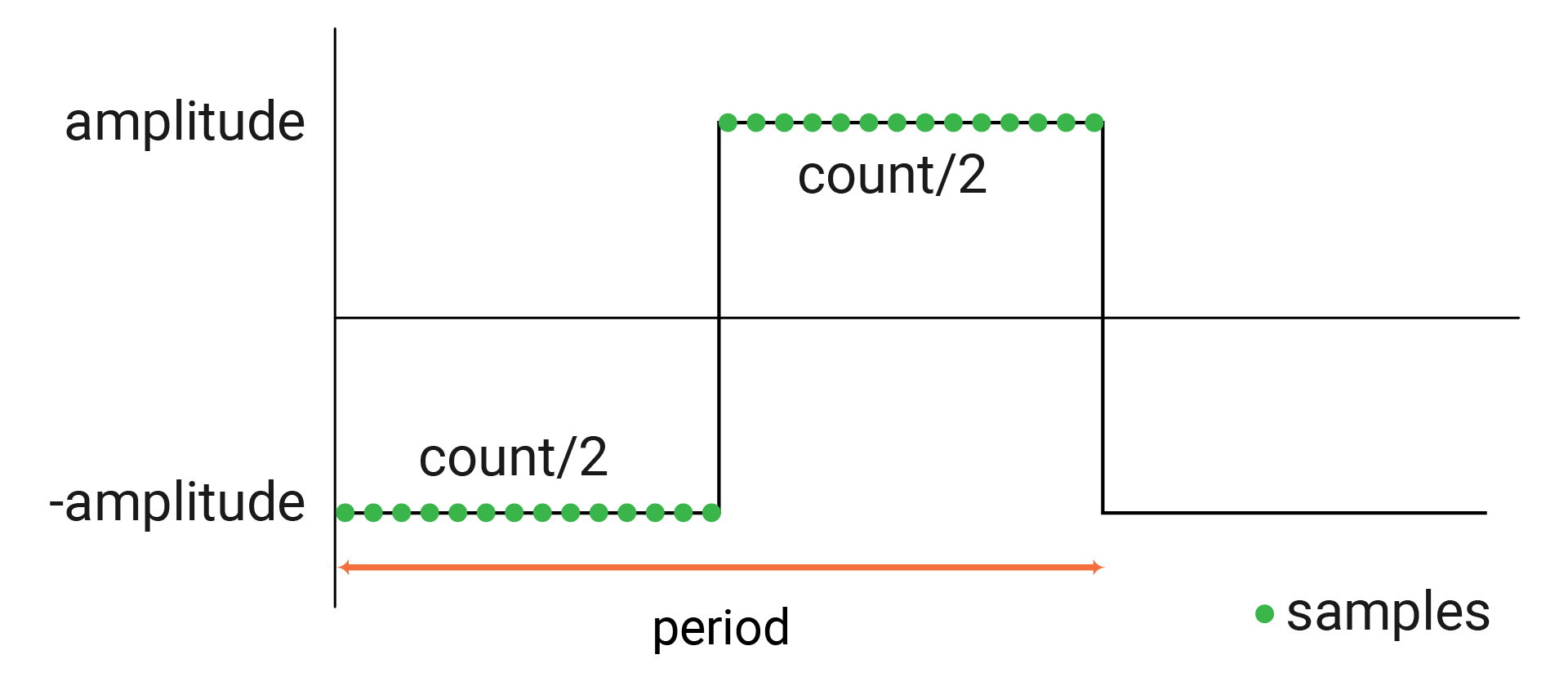``func generateTriangle(amplitude: Int16, _ samples: inout [Int16]) {    let count = samples.count    let step = Float(amplitude) / Float(count / 2)    for i in 0..<count / 4 {        samples[i] = Int16(step * Float(i))    }    for i in count / 4..<count / 4 * 3 {        samples[i] = amplitude - Int16(step * Float(i))    }    for i in count / 4 * 3..<count {        samples[i] = -amplitude + Int16(step * Float(i))    }}``

This function is used to generate samples for a triangle wave in a period. The constant `count` is the total of audio samples. The `step` is the change between two continuous samples.

The samples change linearly and are divided into three parts:

• At first, the samples gradually increase to the maximum (`amplitude`).
• In the second part, the samples decrease from the maximum (`amplitude`) to the minimum (minus `amplitude`).
• In the third part, the samples go up from the minimum (minus `amplitude`).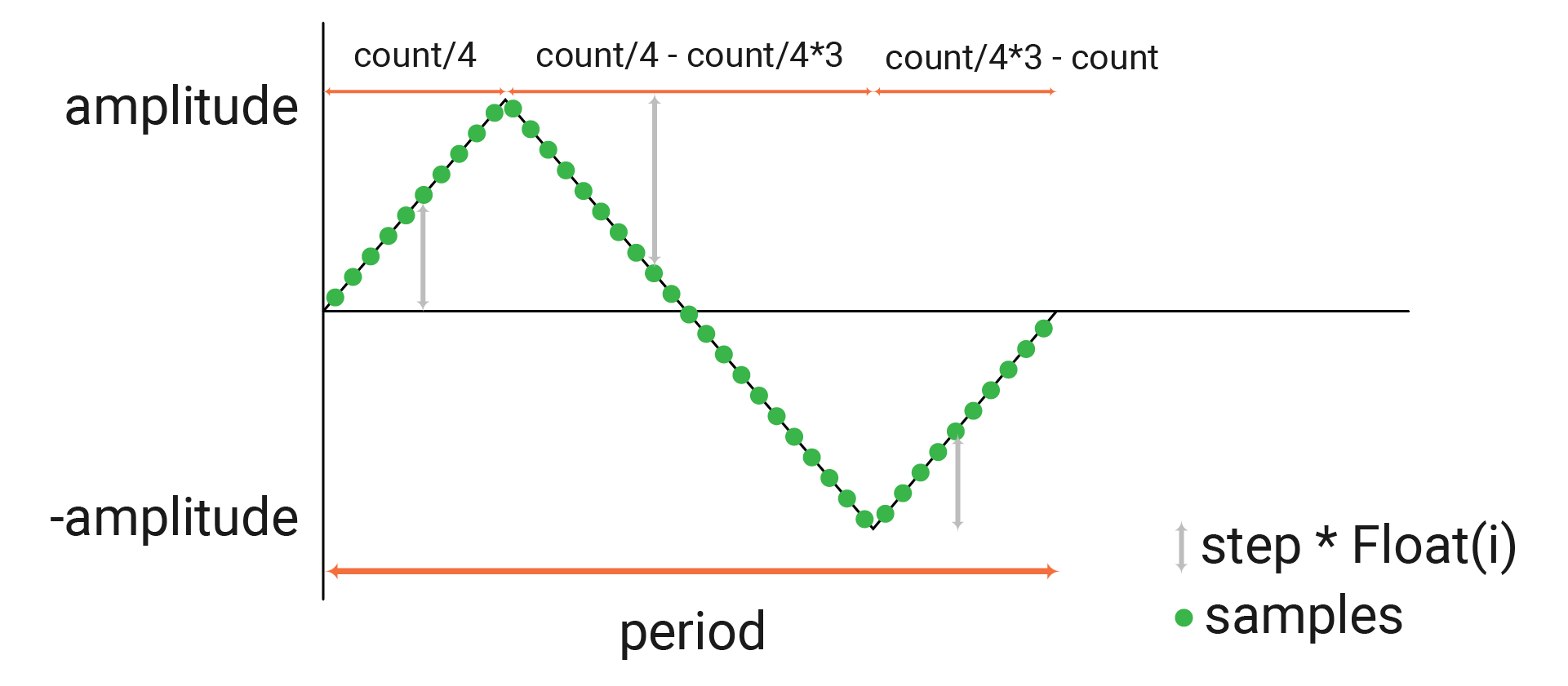``func playWave(samples: [Int16], frequency: Float, duration: Float) {    let playCount = Int(duration * Float(sampleRate))    var data = [Int16](repeating: 0, count: playCount)    let step: Float = frequency * Float(samples.count) / Float(sampleRate)    var volume: Float = 1.0    let volumeStep = 1.0 / Float(playCount)    for i in 0..<playCount {        let pos = Int(Float(i) * step) % samples.count         data[i] = Int16(Float(samples[pos]) * volume)        volume -= volumeStep    }    data.withUnsafeBytes { ptr in        let u8Array = ptr.bindMemory(to: UInt8.self)        speaker.write(Array(u8Array))    }}``

This function sends the samples to audio devices over an I2S bus.

• `playCount` calculates the total amount of samples. `sampleRate` is the amount of samples in 1s, and `duration` is a specified time in seconds. If the note duration is 2 seconds and the sample rate is 16000Hz, the sample count equals 32000.

• The array `data` is used to store the audio data for the speaker. All elements are 0 by default, whose count equals the count of samples calculated before.

• To better understand the constant `step`, assuming a square wave that has 20 samples in a period. Its frequency is 2Hz. Therefore, there will be 40 samples in total in one second. If the audio sample rate is at 10Hz, it needs only 10 samples in one second. So you can choose some of the samples: 1 sample every 4 samples, like samples, samples... So the step here is 20 * 2 / 10 = 4.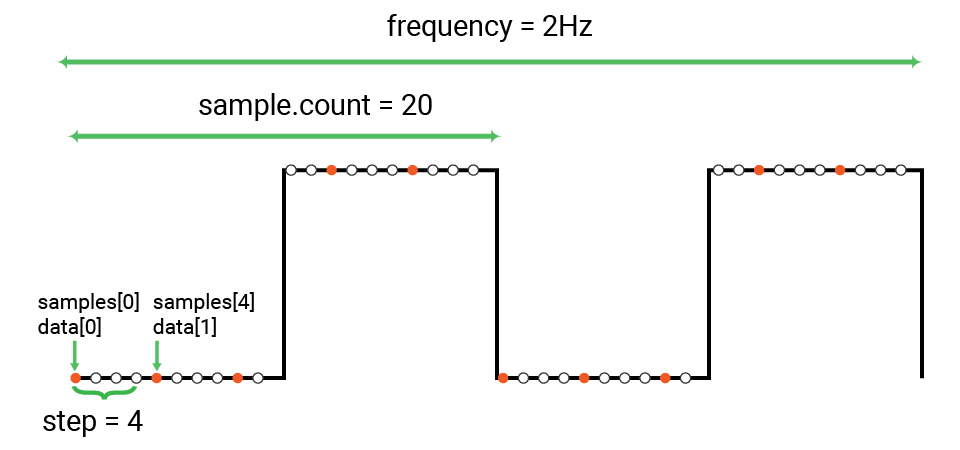• `volume` and `volumeStep` are used to reduce the volume of each note, so it sounds more natural. You could delete the statement `volume -= volumeStep` and see how it sounds. If the `playCount` is 10, the `volume` will be 1, 0,9, 0.8... for each data to fade out the sound.

• In the `for-in` loop, you will store the desired samples into the array `data`. `pos` gets the index of the sample in `samples`. In the wave above, the `pos` is 0, 4, 8, 12, 16. When `pos` equals 20, it refers to the first sample in the next period. The samples are the same with those in the first peropd, so it restarts from 0. After the samples are multiplied by volume, you get gradually decreased sound.

• Send the data using I2S communication so that the speaker plays the note.

``while true {    let duration: Float = 1.0    generateSquare(amplitude: amplitude, &rawSamples)    for f in frequency {        playWave(samples: rawSamples, frequency: f, duration: duration)    }     sleep(ms: 1000)    generateTriangle(amplitude: amplitude, &rawSamples)    for f in frequency {        playWave(samples: rawSamples, frequency: f, duration: duration)    }     sleep(ms: 1000)    amplitude -= 1000    if amplitude <= 0 {        amplitude = 10_000    }}``

In the while loop, the speaker will play scales over and over again.

• At first, the samples are generated from a square wave. Then use these samples to play a scale. So the sound is like what you hear from a buzzer.
• After that, the samples are from a triangle wave. So the sound is softer and clearer.
• The `amplitude` decreases to turn down the speaker. The sound will be lower after each while loop until it reaches the minimum. Then `amplitude` increase to the maximum and repeats the variation.